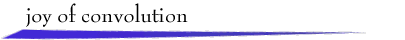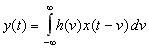Welcome!

The behavior of a linear, continuous-time, time-invariant system with input signal x(t) and output signal y(t) is described by the convolution integralThe signal h(t), assumed known, is the response of the system to a unit impulse input.

To compute the output y(t) at a specified t, first the integrand h(v)x(t - v) is computed as a function of v.Then integration with respect to v is performed, resulting in y(t).

These mathematical operations have simple graphical interpretations.First, plot h(v) and the "flipped and shifted" x(t - v) on the v axis, where t is fixed. Second, multiply the two signals and compute the signed area of the resulting function of v to obtain y(t). These operations can be repeated for every value of t of interest.

To explore graphical convolution, select signals x(t) and h(t) from the provided examples below,or use the mouse to draw your own signal or to modify a selected signal. Then click at a desired value of t on the first v axis. After a moment, h(v) and x(t - v) will appear. Drag the t symbol along the v axis to change the value of t. For each t, the corresponding integrand h(v)x(t - v) and the output value y(t) will be displayed in their respective windows.

 You need a Java-compatible browser to see the demo.return to demonstrations page

 Applet by Steve Crutchfield